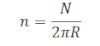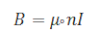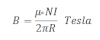# Magnetic Field Due To Toroid

A toroid is a long solenoid which is bend into circular form. So, toroid is equivalent to the solenoid having infinite length but it has finite no. of circular turns. Consider a toroid having radius r, carrying current I through it. If N is the number of turns in toroid and n be the no. of turns per unit length then,again magnetic field due to infinite solenoid is,then the magnetic field due to toroid is,